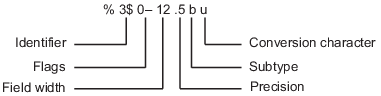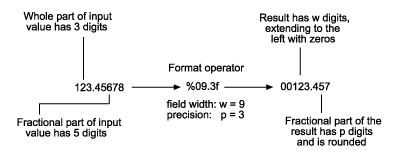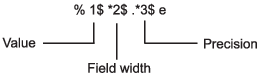## 格式化文本

```A = pi*ones(1,3); txt = sprintf('%f | %.2f | %12f', A)```
```txt = '3.141593 | 3.14 | 3.141593'```

`txt = sprintf('Displaying pi: \n %f \n %.2f \n %12f', A)`
```txt = 'Displaying pi: 3.141593 3.14 3.141593'```

### 格式化操作符的字段#### 转换字符

`c`

`d`

`e`

`E`

`f`

`g`

`G`

`%g` 相同，但使用大写 `E`

`o`

`s`

`u`

`x`

`X`

```A = 46*ones(1,4); txt = sprintf('%d %f %e %X', A)```
```txt = '46 46.000000 4.600000e+01 2E'```

#### 子类型

 `b` 输入数据为双精度浮点值，而不是无符号整数。例如，要以十六进制格式输出双精度值，请使用类似 `%bx` 的格式。 `t` 输入数据为单精度浮点值，而不是无符号整数。

#### 精度

`txt = sprintf('%g %.2g %f %.2f', pi*50*ones(1,4))`
```txt = '157.08 1.6e+02 157.079633 157.08'```

#### 字段宽度

`txt = sprintf('|%e|%15e|%f|%15f|', pi*50*ones(1,4))`
```txt = '|1.570796e+02| 1.570796e+02|157.079633| 157.079633|'```

`txt = sprintf('%30s', 'Pad left with spaces')`
```txt = ' Pad left with spaces'```

#### 标志

`%-5.2d`

`%+5.2d`
`%+5s`

`% 5.2f`

`%05.2f`

• 对于 `%o``%x``%X`，将输出 `0``0x``0X` 前缀。

• 对于 `%f``%e``%E`，即使精度为零也将输出小数点。

• 对于 `%g``%G`，不删除尾随零或小数点。

`%#5.0f`

```txt = sprintf('right-justify: %12.2f\nleft-justify: %-12.2f',... 12.3, 12.3)```
```txt = 'right-justify: 12.30 left-justify: 12.30 '```

```txt = sprintf('no sign: %12.2f\nsign: %+12.2f',... 12.3, 12.3)```
```txt = 'no sign: 12.30 sign: +12.30'```

```txt = sprintf('Pad with spaces: %12.2f\nPad with zeroes: %012.2f',... 5.2, 5.2)```
```txt = 'Pad with spaces: 5.20 Pad with zeroes: 000000005.20'```

#### 值标识符

```sprintf('%s %s %s',... '1st','2nd','3rd') ans = '1st 2nd 3rd' ```
```sprintf('%3\$s %2\$s %1\$s',... '1st','2nd','3rd') ans = '3rd 2nd 1st' ```

`''`

`%%`

`\\`

`\a`

`\b`

`\f`

`\n`

`\r`

`\t`

`\v`

`\xN`

`\N`

### 设置字段宽度和精度

#### 精度和字段宽度的格式化规则• 如果未指定精度，则精度默认为 6。

• 如果精度 `p` 小于输入项的小数部分的位数，则在小数点后只会显示 `p` 位数。输出项中的小数值将被舍入。

• 如果精度 `p` 大于输入项的小数部分的位数 `f`，则在小数点后会显示 `p` 位数。输出项中的小数部分将使用 `p-f` 个零向右侧扩充。

• 如果未指定字段宽度，则字段宽度默认为 `p+1+n`，其中 `n` 为输入值的整数部分的位数。

• 如果字段宽度 `w` 大于 `p+1+n`，则输出值的整数部分将使用 `w-(p+1+n)` 个其他字符向左侧填充。其他字符为空格字符，除非格式化操作符包含 `0` 标志。在这种情况下，其他字符为零。

#### 在格式设定符外部指定字段宽度和精度

```txt = sprintf('%*f %.*f %*.*f',... 15,123.45678,... 3,16.42837,... 6,4,pi)```
```txt = ' 123.456780 16.428 3.1416'```

`%*f`

`%.*f`

`%*.*f`

`txt = sprintf('%*.2f', 5, 123.45678)`
```txt = '123.46'```

#### 在宽度和精度字段中指定带编号的标识符```txt = sprintf('%1\$*4\$f %2\$.*5\$f %3\$*6\$.*7\$f',... 123.45678, 16.42837, pi, 15, 3, 6, 4)```
```txt = ' 123.456780 16.428 3.1416'```

`%1\$*4\$f`

`1\$` 将第一个输入参数 `123.45678` 指定为值

`*4\$` 将第四个输入参数 `15` 指定为字段宽度

`%2\$.*5\$f`

`2\$` 将第二个输入参数 `16.42837` 指定为值

`.*5\$` 将第五个输入参数 `3` 指定为精度

`%3\$*6\$.*7\$f`

`3\$` 将第三个输入参数 `pi` 指定为值

`*6\$` 将第六个输入参数 `6` 指定为字段宽度

`.*7\$` 将第七个输入参数 `4` 指定为精度

### 使用标识符的限制

```sprintf('%d %d %d %d',... 1,2,3,4) ans = '1 2 3 4' ```
```sprintf('%d %3\$d %d %d',... 1,2,3,4) ans = '1 ' ```

```sprintf('%d',1,2,3,4) ans = '1234' ```
```sprintf('%1\$d',1,2,3,4) ans = '1' ```

```v = [1.4 2.7 3.1]; sprintf('%.4f %.4f %.4f',v) ans = '1.4000 2.7000 3.1000' ```
```v = [1.4 2.7 3.1]; sprintf('%3\$.4f %1\$.4f %2\$.4f',v) ans = 1×0 empty char array ```